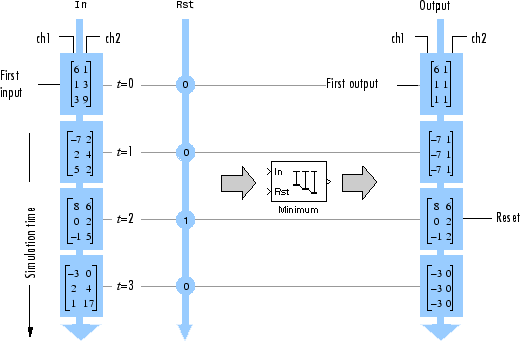# Compute the Running Minimum

Compute the running minimum of a 3-by-2 matrix input, `dsp_examples_u`, using the Minimum block.

Open the model.

```model = 'ex_runningminimum_ref'; open_system(model) ```The Input processing parameter is set to `Columns as channels (frame based)`. The block processes the input as a two-channel signal with a frame size of three. The running minimum is reset at t = 2 by an impulse to the block's Rst port.

Run the model.

```sim(model) ```In the `Running` mode, the block outputs the minimum value over each channel since the last reset. At t = 2, the reset event occurs. The minimum value in the second column changes to 6, and then 2, even though these values are greater than 1, which was the minimum value since the previous reset event.

Close the model.

```close_system(model) ```